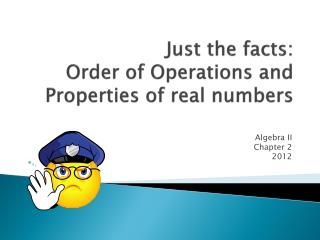# Just the facts: Order of Operations and Properties of real numbers - PowerPoint PPT PresentationDownload PresentationJust the facts: Order of Operations and Properties of real numbers

Just the facts: Order of Operations and Properties of real numbersDownload Presentation## Just the facts: Order of Operations and Properties of real numbers

- - - - - - - - - - - - - - - - - - - - - - - - - - - E N D - - - - - - - - - - - - - - - - - - - - - - - - - - -
##### Presentation Transcript

1. Just the facts: Order of Operations and Properties of real numbers Algebra II Chapter 2 2012

2. Important things toremember • Parenthesis – anything grouped… including information above or below a fraction bar. • Exponents – anything in the same family as a ‘power’… this includes radicals (square roots). • Multiplication- this includes distributive property (discussed in detail later). Some items are grouped!!! • Multiplication and Division are GROUPED from left to right (like reading a book- do whichever comes first. • Addition and Subtraction are also grouped from left to right, do whichever comes first in the problem.

3. So really it looks like this….. • Parenthesis • Exponents • Multiplication and Division • Addition and Subtraction In order from left to right In order from left to right

4. SAMPLE PROBLEM #1 Parenthesis Exponents This one is tricky! Remember: Multiplication/Division are grouped from left to right…what comes 1st? Division did…now do the multiplication (indicated by parenthesis) More division Subtraction

5. SAMPLE PROBLEM Exponents Parenthesis Remember the division symbol here is grouping everything on top, so work everything up there first….multiplication Division – because all the work is done above and below the line Subtraction

6. Order of Operations-BASICSThink: PEMDAS Please Excuse My Dear Aunt Sally • Parenthesis • Exponents • Multiplication • Division • Addition • Subtraction

7. Lesson Extension • Can you fill in the missing operations? • 2 - (3+5) + 4 = -2 • 4 + 7 * 3 ÷ 3 = 11 • 5 * 3 + 5 ÷ 2 = 10

8. Part 2: Properties of Real Numbers(A listing) • Associative Properties • Commutative Properties • Inverse Properties • Identity Properties • Distributive Property All of these rules apply to Addition and Multiplication

9. Associative PropertiesAssociate = group Rules: Associative Property of Addition (a+b)+c = a+(b+c) Associative Property of Multiplication (ab)c = a(bc) It doesn’t matter how you group (associate) addition or multiplication…the answer will be the same! Samples: Associative Property of Addition (1+2)+3 = 1+(2+3) Associative Property of Multiplication (2x3)4 = 2(3x4)

10. Commutative PropertiesCommute = travel (move) Rules: Commutative Property of Addition a+b = b+a Commutative Property of Multiplication ab = ba It doesn’t matter how you swap addition or multiplication around…the answer will be the same! Samples: Commutative Property of Addition 1+2 = 2+1 Commutative Property of Multiplication (2x3) = (3x2)

11. Stop and think! • Does the Associative Property hold true for Subtraction and Division? • Does the Commutative Property hold true for Subtraction and Division? Is (5-2)-3 = 5-(2-3)? Is (6/3)-2 the same as 6/(3-2)? Is 5-2 = 2-5? Is 6/3 the same as 3/6? Properties of real numbers are only for Addition and Multiplication

12. Inverse PropertiesThink: Opposite Rules: Inverse Property of Addition a+(-a) = 0 Inverse Property of Multiplication a(1/a) = 1 What is the opposite (inverse) of addition? What is the opposite of multiplication? Subtraction (add the negative) Division (multiply by reciprocal) Samples: Inverse Property of Addition 3+(-3)=0 Inverse Property of Multiplication 2(1/2)=1

13. Identity Properties Rules: Identity Property of Addition a+0 = a Identity Property of Multiplication a(1) = a What can you add to a number & get the same number back? What can you multiply a number by and get the number back? 0 (zero) 1 (one) Samples: Identity Property of Addition 3+0=3 Identity Property of Multiplication 2(1)=2

14. Distributive Property If something is sitting just outside a set of parenthesis, you can distribute it through the parenthesis with multiplication and remove the parenthesis. Rule: a(b+c) = ab+bc • Samples: • 4(3+2)=4(3)+4(2)=12+8=20 • 2(x+3) = 2x + 6 • -(3+x) = -3 - x